# Tips to reduce Python object size

• Last Updated : 24 Apr, 2020

We all know a very common drawback of Python when compared to programming languages such as C or C++. It is significantly slower and isn’t quite suitable to perform memory-intensive tasks as Python objects consume a lot of memory. This can result in memory problems when dealing with certain tasks. When the RAM becomes overloaded with tasks during execution and programs start freezing or behaving unnaturally, we call it a Memory problem.

Let’s look at some ways in which we can use this memory effectively and reduce the size of objects.

## Using built-in Dictionaries:

We all are very familiar with dictionary data type in Python. It’s a way of storing data in form of keys and values. But when it comes to memory management, dictionary isn’t the best. In fact, it’s the worst. Let’s see this with an example:

 `# importing the sys library``import` `sys `` ` `Coordinates ``=` `{``'x'``:``3``, ``'y'``:``0``, ``'z'``:``1``}`` ` `print``(sys.getsizeof(Coordinates))`
Output:
```288
```

We see that one instance of the data type dictionary takes 288 bytes. Hence it will consume ample amount of memory when we will have many instances: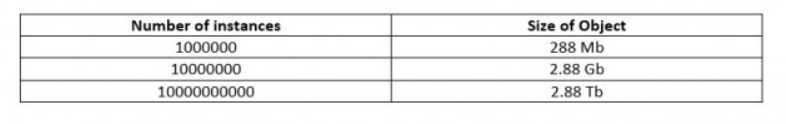So, we conclude that dictionary is not suitable when dealing with memory-efficient programs.

## Using tuples:

Tuples are perfect for storing immutable data values and is also quite efficient as compared to dictionary in reducing memory usage:

 `import` `sys`` ` `Coordinates ``=` `(``3``, ``0``, ``1``)`` ` `print``(sys.getsizeof(Coordinates))`
Output:
```72
```

For simplicity, we assumed that the indices 0, 1, 2 represent x, y, z respectively. So from 288 bytes, we came down to 72 bytes by just using tuple instead of dictionary. Still it’s not very efficient. If we have large number of instances, we would still require large memory: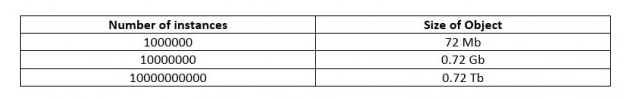## Using class:

By arranging the code inside classes, we can significantly reduce memory consumption as compared to using dictionary and tuple.

 `import` `sys`` ` `class` `Point:`` ` `    ``# defining the coordinate variables``    ``def` `__init__(``self``, x, y, z):``        ``self``.x ``=` `x``        ``self``.y ``=` `y``        ``self``.z ``=` `z`` ` `Coordinates ``=` `Point(``3``, ``0``, ``1``)``print``(sys.getsizeof(Coordinates))`
Output:
```56
```

We see that the same program now requires 56 bytes instead of the previous 72 bytes. The variables x, y and z consume 8 bytes each while the rest 32 bytes are consumed by the inner codes of Python. If we have a larger number of instances, we have the following distribution –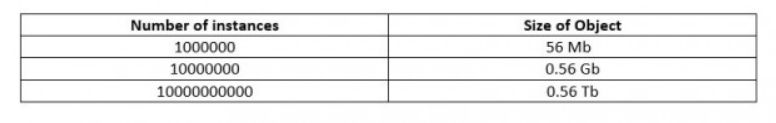So we conclude that classes have an upper-hand than dictionary and tuple when it comes to memory saving.

Side Note : Function sys.getsizeof(object[, default]) specification says: “Only the memory consumption directly attributed to the object is accounted for, not the memory consumption of objects it refers to.”

 `class` `Point:`` ` `    ``def` `__init__(``self``, x, y, z):``        ``self``.x ``=` `x``        ``self``.y ``=` `y``        ``self``.z ``=` `z`` ` `Coordinates ``=` `Point(``3``, ``0``, ``1``)`

the effective memory usage of object Coordinates is:
sys.getsizeof(Coordinates) +
sys.getsizeof(Coordinates.x) +
sys.getsizeof(Coordinates.y) +
sys.getsizeof(Coordinates.z) =
= 56 + 28 + 24 + 28 =
= 136

## Using recordclass:

`Recordclass` is a fairly new Python library. It comes with the support to record types which isn’t in-built in Python. Since `recordclass `is a third-party module licensed by MIT, we need to install it first by typing this into the terminal:

`pip install recordclass`

Let’s use `recordclass `to see if it further helps in reducing memory size.

 `# importing the installed library``import` `sys``from` `recordclass ``import` `recordclass `` ` `Point ``=` `recordclass(``'Point'``, (``'x'``, ``'y'``, ``'z'``))`` ` `Coordinates ``=` `Point(``3``, ``0``, ``1``)``print``(sys.getsizeof(Coordinates))`

Output:

`48`

So the use of `recordclass `further reduced the memory required of one instance from 56 bytes to 48 bytes. This will be the distribution if we have large number of instances: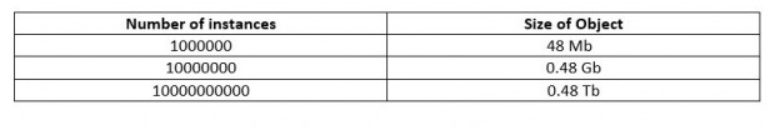## Using dataobjects:

In previous example, while using `recordclass`, even the garbage values are collected thus wasting unnecessary memory. This means that there is still a scope of optimization. That’s exactly were dataobjects come in use. The dataobject functionality comes under the recordclass module with a specialty that it does not contribute towards any garbage values.

 `import` `sys``from` `recordclass ``import` `make_dataclass`` ` `Position ``=` `make_dataclass(``'Position'``, (``'x'``, ``'y'``, ``'z'``))``Coordinates ``=` `Position(``3``, ``0``, ``1``)`` ` `print``(sys.getsizeof(Coordinates))`

Output:

`40`

Finally, we see a size reduction from 48 bytes per instance to 40 bytes per instance. Hence, we see that dataobjects are the most efficient way to organize our code when it comes to least memory utilization.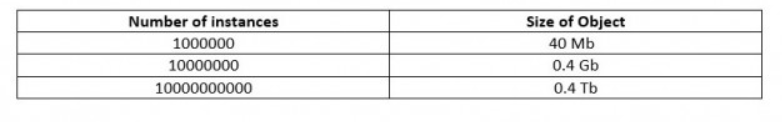My Personal Notes arrow_drop_up